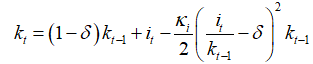# Questions on solving the model

Dear Professor Pfeifer,

I was puzzled by the problems as follows when trying to solve the DSGE model. Would you please give any advice? Thank you for your time.

(1) When I use averages of empirical data(e.g. C/Y, I/Y) to do the calibration in DSGE model with no trend, do I need to eliminate the growth rate of these data series(e.g. Y, C, I ) respectively? I divide raw quarterly data series by quarter-on-quarter growth, is this correct?

And also, before I deal with these data series for the bayesian estimation, do I also need to eliminate the growth rate ?

(2) When comparing the second moments of variables between model and empirical data to check the model fit, do I need to confirm all major variables fit well with empirical data? I read in some papers that not all the moments of empirical data perfectly lie in the interval that the model implies. Does this mean that it is acceptable to allow the error in between? Will this impact the reliability of simulated results ?

(3) I want to add investment shock into capital accumulation function, and I read the formation likeor. How to decide which one should be chosen?
And what about the function like? Is it correct to add investment shock like?

1. I am not entirely sure what you mean. What do you mean with

Taking out the mea, i.e. working with mean 0 growth rates? And what do you mean with

? Why would you divide a level by a growth rate?
2. See How to see whether the model fit the real data well?

Dear Professor Pfeifer,

(1) I read in a book that I should eliminate the trend term of data series before using them to calibrate the parameters in a dsge model with no trend. Is this correct ? And how should I realize this ?

(2) Sorry but I did not find the answer in that post. Maybe I should make it more clear. For instance, the std of output variable Y that the model implied is between 1.5 and 2.5 while the std of empirical data Y is 2.8 or sth out of the interval that model implied. Do I need to confirm all the stds or cross correlations of major variables fit well with empirical data?
I read in some papers that not all these features of empirical data perfectly lie in the model-implied interval. Does this mean that it is acceptable to allow the error in between ? And to what extend will this impact the reliability of simulated results ?

(3) Sorry and I rewrite the question:
I want to add investment shock into capital accumulation function, and I read the formation like
{{k}_{t}}=\left( 1-\delta \right){{k}_{t-1}}+\varepsilon _{t}^{i}\left[ 1-\frac{{{\kappa }_{i}}}{2}{{\left( \frac{{{i}_{t}}}{{{i}_{t-1}}}-1 \right)}^{2}} \right]{{i}_{t}}
or
{{k}_{t}}=\left( 1-\delta \right){{k}_{t-1}}+\left[ 1-\frac{{{\kappa }_{i}}}{2}{{\left( \frac{\varepsilon _{t}^{i}{{i}_{t}}}{{{i}_{t-1}}}-1 \right)}^{2}} \right]{{i}_{t}}.
How to decide which one should be chosen?

And what about the accumulation function like
{{k}_{t}}=\left( 1-\delta \right){{k}_{t-1}}+{{i}_{t}}-\frac{{{\kappa }_{i}}}{2}{{\left( \frac{{{i}_{t}}}{{{k}_{t-1}}}-\delta \right)}^{2}}{{k}_{t-1}} ?
Is it correct to add investment shock as
{{k}_{t}}=\left( 1-\delta \right){{k}_{t-1}}+{{i}_{t}}-\frac{{{\kappa }_{i}}}{2}{{\left( \frac{\varepsilon _{t}^{i}{{i}_{t}}}{{{k}_{t-1}}}-\delta \right)}^{2}}{{k}_{t-1}} ?